## SAO Guest ContributionHow to Simulate the Universe in a Computer
Dr. Alexander Knebe
Centre for Astrophysics and Supercomputing
Swinburne University of Technology.

Alexander Knebe is a newly arrived postdoctoral fellow at the Centre for Astrophysics and Supercomputing. He works on cosmological simulations of the evolution of the Universe, and in this presentation he explains how such simulations are being preformed using modern compuational techniques. Alexander spent the last two years at Oxford Unviversity developing one of the few adaptive grid-based codes for such tasks. He completed his PhD theis on "The formation and evolution of galaxy clusters within the large scale structure of the Universe" at Astrophyscial Institute Potsdam in Germany.

### Introduction

The purpose of a cosmological simulation is to model the growth of structures in the Universe and have a long history and numerous applications. These simulations play a very significant role in cosmology because they are the one and only 'experiment' to verify theories of the origin and evolution of the Universe.

The Universe is expected to have started with a Big Bang in which - or more precisely: shortly afterwards - tiny fluctuations were imprinted into the radiation and matter density field (in the otherwise homogeneous and isotropic Universe). To understand how the Universe evolved from that early stage into what we observe today (stars, galaxies, galaxy clusters, ...) one needs to follow the non-linear evolution of those density fields using numerical methods.

So, the process of a cosmological simulation is actually twofold: one first needs to generate some initial conditions according to the structure formation model one likes to investigate, which are then used as an input to the code itself. In all codes the evolution is simulated by following the trajectories of particles under their mutual gravity. And the law of gravity is simply just the Newtonian force law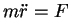where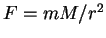(the 'r with two dots' is just another way of writing the second derivative of r, ie. d2r/dt2, which is just the acceleration).

These particles are supposed to sample the matter density field as accurately as possible and a cosmological simulation is nothing more (and nothing less) than a simple and effective tool for investigating the evolution of millions of particles. However, there are two constraints on a cosmological simulation, (a) the correct initial conditions and (b) the correct final particle distribution which ought to agree with observations of the Universe as seen today. In Fig. 1 you can find a rough sketch of the cosmic evolution the Universe went through and simulations are trying to bridge the gap between observations of the early Universe (i.e. anisotropies in the Cosmic Microwave Background observed as early as 300000 years after the Big Bang) and the modern Universe.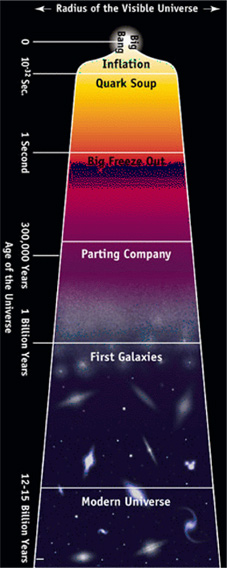The first application of numerical simulations in astrophysics was the simulation of star clusters  using as little as a couple of hundred particles. During the 1970's more simulations of galaxy clustering were performed using what is called PP (particle-particle) methods  and finally in 1981 the first cosmological simulations using more than 20000 particles appeared . However, already those simulations were able to make credible predictions and the problems showing up in them remain unsolved even when using the latest high resolution simulations, i.e., the steep rise of the density profile of galaxies in the very central parts predicted by the simulations which is not matched by observation.

It is necessary to use as many particles as possible because the idea always is that the particles are sampling the density of the Universe rather than being individuals. This is different to using numerical simulations for modelling the formation of globular clusters where each particle represents a single star. In a cosmological simulations the particles are not to be confused with galaxies, stars, or whatever. They are more to be understood as sampling points of a fluid/density field that moves under its own gravity. But the problem is that the more particles used, the more computer time a simulation consumes. And even with the largest supercomputers nowadays it is only possible to use some million particles if we want to retrieve results in a reasonable time (say, couple of weeks). And until now the methods for simulating millions of gravitationally interacting particles have been continiously refined to allow for more and more particles to be used while simultaneously resolving finer and finer structures. These simulations can resolve the orbits of dwarfs galaxies (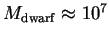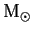) within galactic haloes (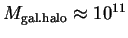) in cosmological volumes of the order (30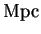)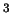.

In this contribution I would like to focus on various numerical techniques, their advantages and their shortcomings when being compared with each other. I need to stress that all those simulations are based on the assumption that the Universe mainly consists of Dark Matter that only interacts via gravitation. Baryonic matter, which only accounts for about 10% of the total mass of the Universe, is completely neglected in these simulations. Only nowadays the methods and the computer technology have become as sophisticated as to allow for hydrodynamical processes (i.e. gas dynamics) to be included in such time consuming calculations, but the field is still in its infancy.

Finally, have a look at Fig. 2 to get an idea of what it looks like to simulate the Universe in a computer.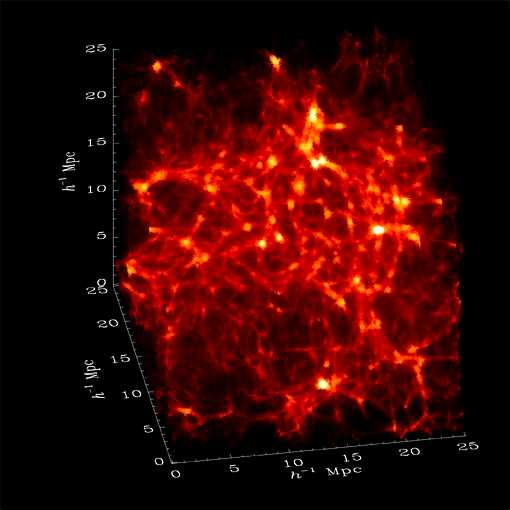### Newtonian Gravity

The whole idea is to use Newton's second law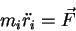(1)

with the correct choice for the force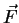. And most of the computational time during a numerical simulation of the Universe is actually spent on deriving the forces at every single particle position.

As already mentioned in the Introduction, each particle feels a force that is given by the Newtonian gravity law: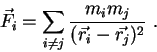(2)

Because we need to take into account the forces from all particles other than particle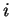the force on this particular particle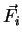is the sum (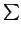) of all the forces from the other particles (as given by Eq. (2)).

However, Eq. (2) is just the special case where we are dealing with point-like masses. But the actual idea is to use the particles as sampling points for the density. Therefore the correct equation to use is not Newton's force law Eq. (2), but the generalisation of it - called Poisson's equation: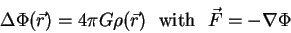(3)

One can see that this equation involves the density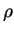and it can be easily proved that the assumption of dealing with point-like masses leads to exactly Eq. (2) for the force. The new quantity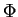introduced in Eq. (3) is called the potential and in the theory of classical mechanics it is a more convenient way of describing gravity to userather than the force vector.

Once we know the potential,we can readily calculate the force at each particle location which is crucial for integrating the equations of motions Eq. (1). The proper way of doing so is going to be outlined later on in the Section on Newtonian Dynamics.

Anyway, their are two different techniques being used for cosmological simulations. The one is based on Eq. (2) and the other on Eq. (3):

 1. direct particle-particle summation as given by Eq. (2)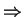Tree Codes (or PP method) 2. numerical integration of Eq. (3) on a gridParticle-Mesh Codes

which will be explained in more detail later on.

### Mass Resolution

I still need to mention that a cosmological simulation in practice only simulates a certain part of the Universe. This is what people refer to as the simulation box or computational box. However, to account for the fact the Universe actually is indefinite one uses periodic boundary conditions: particles leaving the box on the one side are immediately entering the box again on the other side. This is sketched in Fig. 3 which indicates how a particle leaving the right side of the computational box enters again on the left hand side.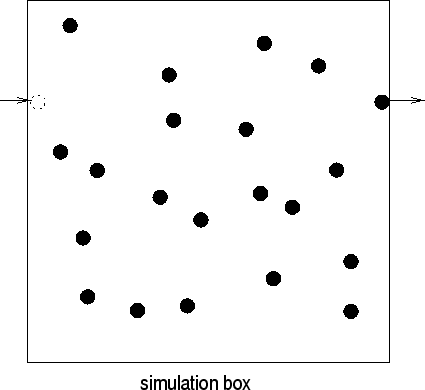Moreover, the size of the simulation box also defines the mass resolution of the simulation. We are only using a certain number of particles within a well defined region of the Universe (the box). And as the denisty of the Universe is given by the cosmological model under investigation, we can calculate the mass of a single particle. This mass determines the mass resolution of that specific simulation. For instance, if we model the evolution of about 2 million particles in a (25)box within a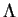CDM model (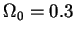), each particle weighs about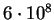. So, we won't be able to resolve dwarf galaxies in that particular cosmological simulation (since).

### Tree Codes - The Forces

The PP (particle-particle) method upon which Tree codes are based assumes to deal with point-like masses and therefore Eq. (2) is applicable. This is also why this technique is called PP: it uses the direct particle-particle summation to calculate the forces.

Because we are interested in deriving the forces at every single particle position, the PP method scales like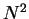: if we double the number of particles it takes four times as much time to actually run the simulation. Therefore this PP method is not feasible for a sufficient number of particles, not even on the largest supercomputers available nowadays ! One needs to come up with a more sophisticated treatment decreasing the need for computer time, and one way of achieving this is to organize the particles in a tree-like structure: if particles are located ''far away'' from the actual particle at which position we intend to calculate the force, these particles can be lumped together as a single but more massive particle at the mean distance (cf. Fig. 4). This decreases the number of calculations dramatically, bringing such codes back into the field of competition with other methods.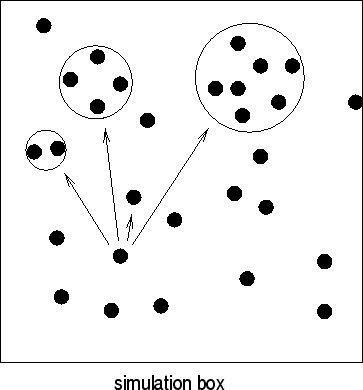One publically available Tree code is called GADGET (Galaxies with Dark Matter and Gas intEracT) written by one of my colleagues Volker Springel . Feel free to download it from the following web page and have a play with it: http://www.mpa-garching.mpg.de/gadget/

### Tree Codes - The Force Resolution

We need to (manually) set a limit on the minimal allowed spatial separation between two particles for which we can actually calculate the forces. This is because of the singularity arising from the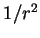force law in Eq. (2) which can be avoided by introducing a (fixed) scale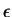. This parameter determines the smallest possible separation for two individual particles: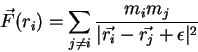(4)

The scale defined by the parameteris usually called the softening parameter or the force resolution of a numerical simulation run by a code which uses a PP summation.

### Particle-Mesh Codes

Another way of deriving the forces is to use Poisson's equation (3) and to numerically integrate it. This approach is closer to the idea of a cosmological simulation which intends to follow the evoution of the density rather than the interaction of individual particles. But this method requires the introduction of a grid on which we can define the density and also solve Eq. (3) numerically. The grid is usually a regular one with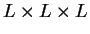cells where each cell is identified by the triple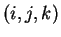with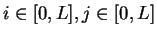and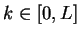(i.e., each of i, j and k run from 0 to L). The itinerary for calculating the force at particle position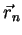due to the denisty field of all particles looks exactly like this:

1.
assign all particles to the grid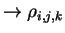2.
solve Poisson's equation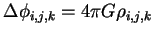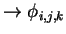3.
differentiate to get forces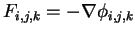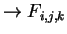4.
interpolate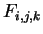back to particle positions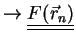In this scheme most of the time is also spent at point 2 and there are various methods for solving Poisson's equation.

### Particle Mesh Codes - The Forces

I do not want to go into the details of how to numerically solve a (partial) diffetential equation. The only thing that needs to be mentioned is that most people use Fourier transformations. On the largest supercomputers in the world there are software libraries available which include the fastest routines for Fourier transformations one can imagine. To actually benchmark supercomputers this is the recognized procedure: the faster the Fourier transform the better the computer. So, most supercomputer manufacturers (i.e. Cray, SGI, IBM, ...) are working extremely hard on selling the world's fastet Fourier transformations along with their machines. And this is why this technique is adopted for solving Poisson's equation as needed in cosmological simulations. However, there are other methods and one of the publically available PM (Particle-Mesh) codes that uses a different integration scheme was written by myself . The code can be downloaded along with exhaustive documentations and some sample cosmological simulations at the following web address:http://www-thphys.physics.ox.ac.uk/users/MLAPM/

### Particle Mesh Codes - The Force Resolution

The problem with the PM method is the lack of spatial resolution below two grid spacings. Whereas in Tree codes one needs to manually fix the problem of the singularity in Eq. (2) for close encounters of particles by introducing the scale(cf. Eq. (4)), in PM we are dealing with sort of the opposite. As gravity tends to clump things together, the particles flow from low density regions into high density regions amplifying primordial density fluctuations. This leads to an excess of particles in certain cells whereas other cells are becoming more and more empty. The PM method can be understood as smoothing the density field (as defined by the particle positions) on a scale determined by the spacing of the grid. Hence particles that are closer than about two cells spacings do not interact according to Eq. (3) with each other. We are left with the situation where we can not resolve structure formation on scales smaller than the cell spacing of the grid !

This is the major problem for PM simulations and the most obvious way to overcome this is to introduce finer grids in such regions. However, these grids needs to freely adapt to the actual particle distribution at all times and hence programming such an adaptive PM method is a very demanding task. Therefore not many research groups took that avenue and there are only a handful of such codes (,,). One of them (and the only one to be publically available) is our very own code MLAPM (Multi-Level-Adaptive-Particle-Mesh) already mentioned.

You can get an idea of the nested grid structure for a radial (two-dimensional) particle distribution with sucessively more and more particles close to the centre in Fig. 5. The upper panel shows the particle positions whereas in the lower panel the adaptive grid structure for solving Poisson's equation is presented.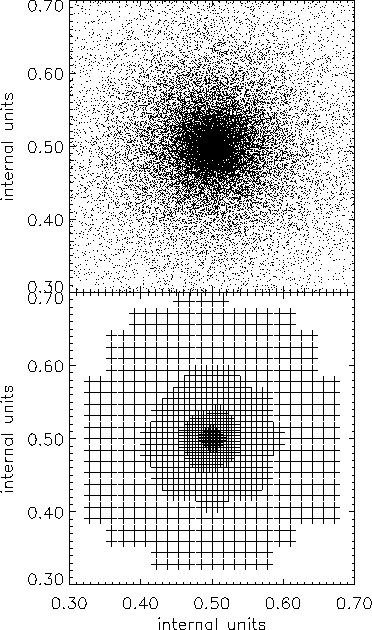Of course there are other ways to increase the force resolution locally and most of my colleagues are using what is called a PM code: a combination of PP and PM methods. Here the force as given by the plain PM calculation is augmented by a direct summation over all neighboring particles within the surrounding cells. This gives accurate forces down to the scale provided by the softening parameteragain.

### Tree vs. Particle-Mesh

The question now arises: which of these methods, Tree or PM, is superior? I already mentioned that the PM technique is actually closer the idea of simulating the evolution of a density field whereas Tree codes are assuming to deal with particles from the very start. Only if we really want to simulate the interplay of individual (point-mass-like) objects (as for instance in a globular cluster, a solar system, or even individual galaxies) one should use Newton's formula for the gravitational force Eq. (1). To simulate the Universe, we want to follow the flow of matter and therefore using the matter densityas a source for gravity seems much more appropriate to me.

In my opinion the problem with Tree as well as with PM codes lies in the possibility of close encounters of two (or more) particles. The formula for the force then becomes singular, because the denominator reaches zero. This problem is fixed by the introduction of a general scale(cf. Eq. (4)). When two particles approach too closely they then undergo a huge acceleration and the time step for integrating the equations of motion (time stepping is going to be discussed in the following Section) might be too small to account for that. Hence they bounce off each other violating the conservation of energy (this effect is called two-body scattering). Moreover, the softening applies to all particles whether they are in a high denity region or a low density region. If we want to resolve structures on kpc-scales in a simulation box with sidelength a couple of Mpc's we need to do so from the very start of the simulation and at every spatial location within that box. But studies have shown that the force resolution should not be better than the local (!) interparticle separation (,,). Codes incorporating a PP direct summation are therefore suffering from a force resolution that is spatially fixed. Low density regions are over-resolved in these codes (which is a waste of computer time as well as leading to artificial effects such as two-body scattering) and high density regions might be affected by two-body collisions.

The introduction of refined grids in PM methods on the other hand guarantees to adapt the force resolution to the actual problem at all times. If we start out with a grid where the number of grid cells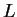per dimension is chosen so that two cell spacings agree with the interparticle separation, we are doing fine. And as soon as particles gather together in certain cells because of the attractive character of gravitation, we split those cells in a manner such that the newly created cells also have a spacing that agrees with the (local) interparticle separation. This procedure is tuned to never ever resolve better than the (local) mean separation length of particles whether the particles are in high or in low density regions.

Anyway, some Tree codes (i.e. GADGET) already take care of problems like the two-body scattering by adapting the time stepping scheme to the local acceleration and hence those authors might claim there is no problem with it at all. Therefore I need to stress that above statements are my personal point of view and this topic is still under hot debate as one needs to make absolutely sure that cosmological simulations are free of numerical artifacts !

### Newtonian Dynamics (in an Expanding Universe)

Last but not least I need to mention the way of updating the positions and velocities of the particles. We are only dealing with Newtonian dynamics and therefore the equation to integrate is simply: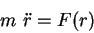(5)

However, the Universe is expanding and it is far more convenient to re-write this equation using what is called comoving coordinates. This is a transformation to a non-inertial (accelerating) frame, but it nevertheless simplifies things. Let us introduce the comoving variable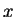: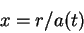(6)

where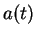is the cosmic expansion factor. Actually calculatingis quite a complicated task because this involves General Relativity. When Einstein wrote down his equations describing gravity, people soon realized from the solutions to those equations that the Universe needs to either expand or collapse (the steady-state Universe was not covered by his orginal equations and was only possible by introducing something like a negative gravitational push). And to calculate the rate of expansion of the Universeone needs to fully solve Einstein's General Relativity equations under certain assumptions (homogeneity and isotropy). However, this calculation is performed during the course of a cosmological simulation and does not consume any of the computational time at all -- it is an easy task once properly programmed.

To time-step a cosmological simulation we need to have the correct initial conditions with the particle positions and velocities given in comoving coordinates at a specific time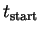. This system is then evolved forward in time until we reach todays time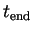whereobviously depends on the cosmological model. The 'gap' betweenand(cf. Fig. 1) is covered by a series of steps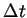which I already refered to as being the time step of the simulation (cf. discussion of Tree vs. Particle Mesh). Early cosmological codes (i.e. APM) use a fixed time step=const. whereas modern codes (i.e. GADGET and MLAPM) also adapt the time step to the actual problem during the course of the simulation. I have to admit that the Tree code GADGET uses a criterion that is based on the local acceleration to match the integration of the equations of motion (Eq. (5) combined with Eq. (6)) to the desired needs for each individual particle. MLAPM only divides the global time step set by the user for the first grid by successive factors of two on the adaptively placed refinements. In any case, particles in high density regions are updated more frequently leading to better accuracy in the time evolution of the simulation.

### Final Remarks

This brief overview of the field of numerical simulations in cosmolgy is only to be understood as a very basic introduction explaining the very basic ideas. If you are interested in this subject there are some recomendable review articles  as well as the one and only textbook on Computer Simulations using Particles .

I haven't covered anything like setting up the initial conditions for a specific cosmological model yet, nor was there any comment on how to actually analyse a simulation. This again would go beyond the idea of this contribution, but feel free to either browse astrophysical journals as Monthly Notices of the Royal Astronomical Society, MNRAS or Astrophysical Journal, ApJ for it or to contact me directly via email (aknebe@astro.swin.edu.au).

### References

  Aarseth S.J., MNRAS 126, 223 (1963)  Peebles P.J.E., Astron. J. 75, 13 (1970)  Efstathiou G., Eastwood J.W., MNRAS 194, 505 (1981)  Ghigna S., Moore B., Governato F., Lake G., Quinn T., Stadel, J., ApJ 544, 616 (2000)  Springel V., Yoshida N., White S.D.M., NewA6512001  Knebe A., Green A., Binney J.J., MNRAS 325, 845 (2001)  Kravtsov A.V., Klypin A.A., Khokhlov A.M., ApJ 111, 73 (1997)  Norman M.L., Bryan G.L., in Numerical Astrophysics, eds. S.Miyama, K.Tomisaka,T.Hanawa (Boston:Kluwer), 1998  Couchman H.M.P., ApJ 368, 23 (1991)  Binney J., Knebe A., astro-ph/0105183  Hamana T., Yoshida N., Suto Y., astro-ph/0111158  Peebles P.J.E., The large-scale structure of the Universe, Princeton University Press, 1980  Bertschinger E., Ann. Rev. A & A 36, 599 (1998)  Hockney R.W., Eastwood J.W., Computer Simulations Using Particles, Bristol: Adam Hilger (1988)